| Daily Market Reports, Market Reports

# Kraken Daily Market Report for September 26 2020

## Overview

• Total trading volume at $90.4 million. Bitcoin traded between$10,650 and $10,770 througout the day. • Today was a good day for savvy investors. Some coins such as Melon (-7.0%) and Cosmos (-4.1%) were significantly outperformed by Gnosis (+15%) and Curve DAO (+14%). • Link’s recent hot streak pumped the brakes today with a -3.9% return over USD. September 26, 2020$90.4M traded across all markets today
Crypto, EUR, USD, JPY, CAD, GBP, CHF, AUD
XBT
$10733. ↑0.32%$42.0M
ETH
$354.17 ↑0.5%$14.8M
$10.324 ↓3.9%$10.2M
USDT
$1.0003 ↓0.01%$7.28M
DOT
$4.3264 ↓2.3%$4.58M
XRP
$0.2416 ↓0.07%$2.42M
$0.0955 ↓1.8%$1.58M
BCH
$221.5 ↑2.2%$1.22M
USDC
$1.0001 ↑0.01%$1.07M
LTC
$46.059 ↑0.01%$1.04M
XTZ
$2.1688 ↓2.1%$1.03M
ALGO
$0.3252 ↑2.1%$971K
TRX
$0.0272 ↑0.27%$647K
DAI
$1.0108 ↑0.12%$576K
XMR
$94.630 ↑1.3%$530K
REP
$15.249 ↑5.6%$522K
OXT
$0.3065 ↑2.6%$493K
KAVA
$2.2262 ↑5.8%$406K
OMG
$3.1005 ↓1.7%$397K
ETC
$5.7509 ↓0.4%$392K
CRV
$1.1144 ↑14%$381K
WAVES
$2.5111 ↑4.9%$356K
ATOM
$4.5991 ↓4.1%$325K
XLM
$0.0738 ↓1.2%$321K
STORJ
$0.3993 ↑9.3%$309K
NANO
$0.8272 ↑7.6%$267K
MLN
$32.400 ↓7.0%$253K
DASH
$69.077 ↑0.7%$234K
EOS
$2.5671 ↑0.8%$231K
SNX
$5.2272 ↑6.2%$207K
GNO
$50.219 ↑15%$163K
COMP
$144.11 ↑3.3%$157K
QTUM
$2.3976 ↑1.5%$144K
SC
$0.0027 ↓0.18%$143K
ZEC
$56.204 ↑2.3%$135K
BAT
$0.2325 ↓0.04%$110K
PAXG
$1872.5 ↓0.22%$110K
ICX
$0.4256 ↑2.9%$92.9K
KNC
$1.0233 ↑4.4%$91.6K
KSM
$35.704 ↓3.3%$84.4K
LSK
$1.2212 ↑1.3%$49.3K
BAL
$15.489 ↓0.5%$42.4K
XDG
$0.0027 ↓0.2%$34.4K
REPV2
$14.330 ↑1.7%$13.6K

#####################. Trading Volume by Asset. ##########################################

The figures below break down the trading volume of the largest, mid-size, and smallest assets. Cryptos are in purple, fiats are in blue. For each asset, the chart contains the daily trading volume in USD, and the percentage of the total trading volume. The percentages for fiats and cryptos are treated separately, so that they both add up to 100%.

Figure 1: Largest trading assets: trading volume (measured in USD) and its percentage of the total trading volume (September 26 2020)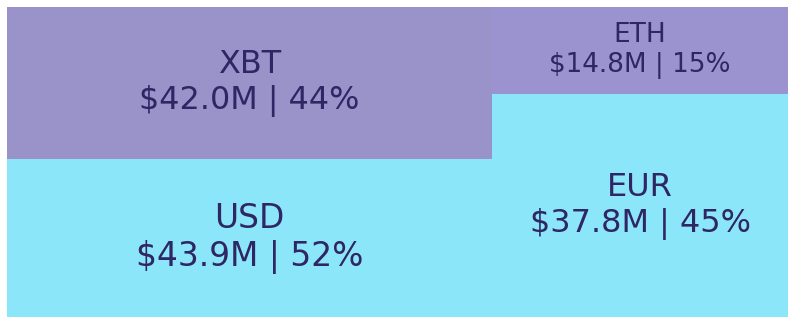Figure 2: Mid-size trading assets: (measured in USD) (September 26 2020)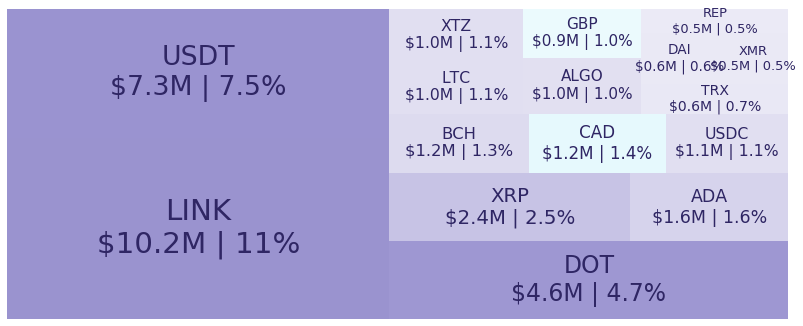Figure 3: Smallest trading assets: (measured in USD) (September 26 2020)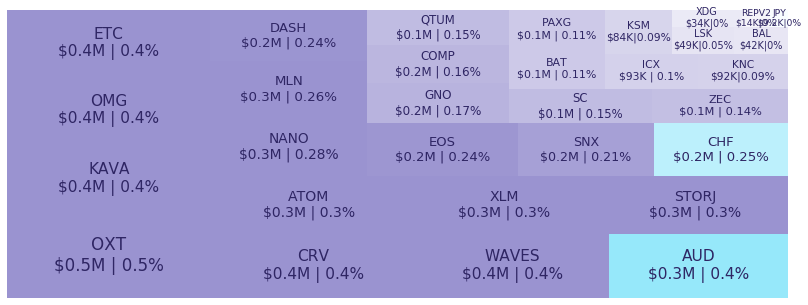Spread percentage is the width of the bid/ask spread divided by the bid/ask midpoint. The values are generated by taking the median spread percentage over each minute, then the average of the medians over the day.

Figure 4: Average spread % by pair (September 26 2020)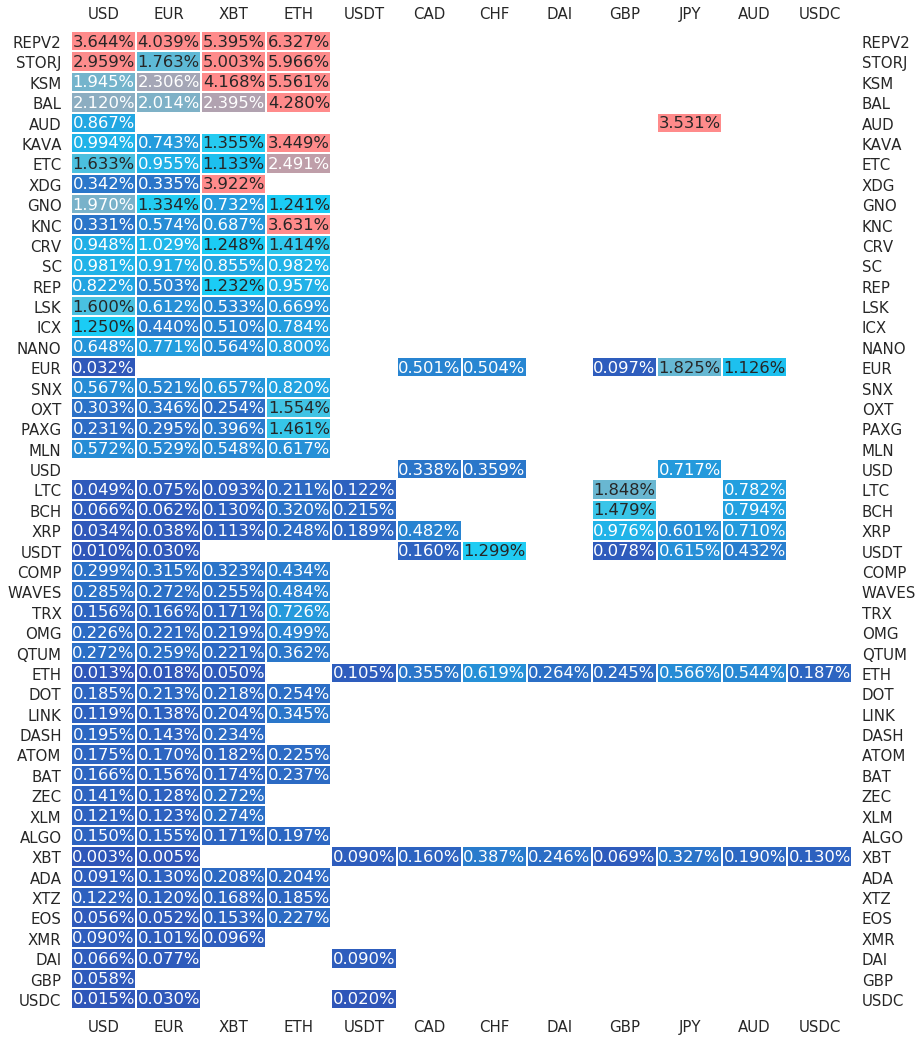.

#########. Returns and Volume ############################################

## Returns and Volume

Figure 5: Returns of the four highest volume pairs (September 26 2020)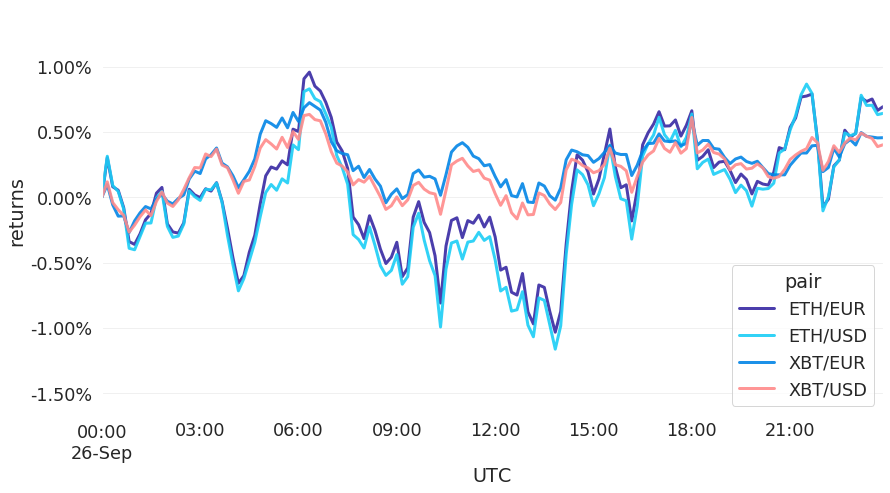Figure 6: Volume of the major currencies and an average line that fits the data to a sinusoidal curve to show the daily volume highs and lows (September 26 2020)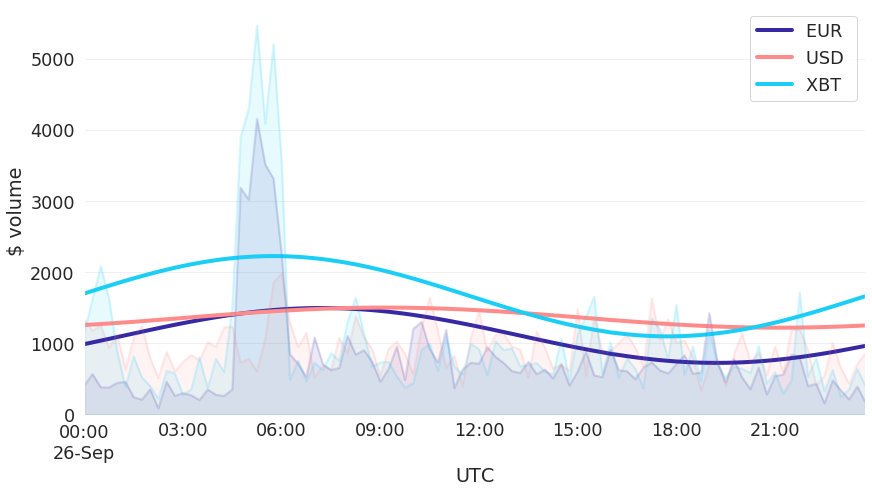###########. Daily Returns. #################################################

## Daily Returns %

Figure 7: Returns over USD and XBT. Relative volume and return size is indicated by the size of the font. (September 26 2020)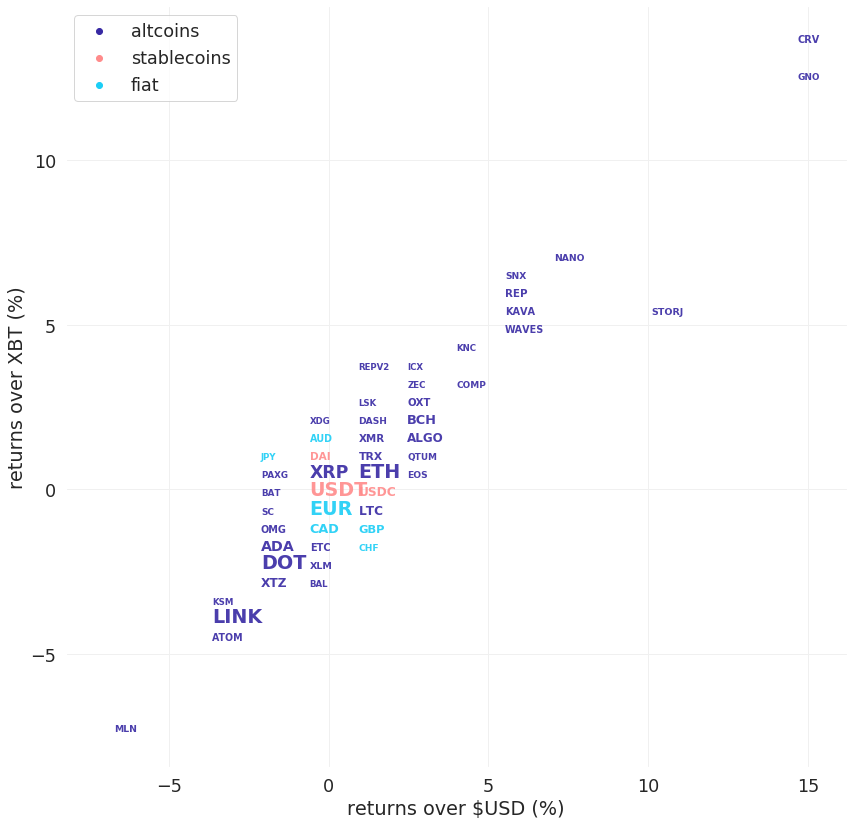###########. Disclaimer #################################################

The values generated in this report are from public market data distributed from Kraken WebSockets api. The total volumes and returns are calculated over the reporting day using UTC time.